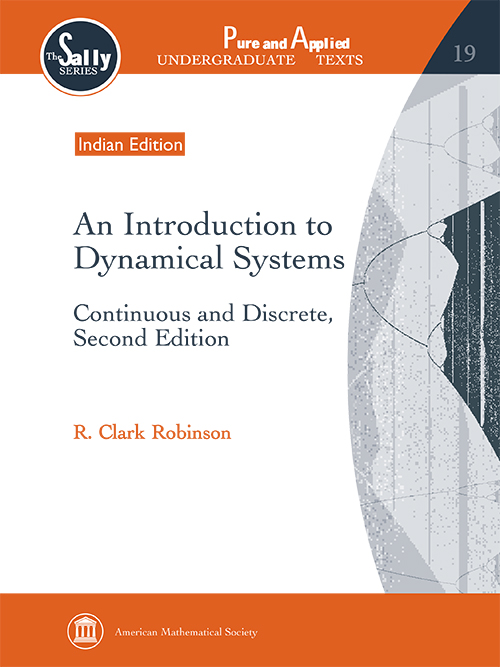Introduction to Dynamical Systems: Continuous and Discrete (Second Edition)
R Clark Robinson
Price
1825.00
ISBN
9781470425807
Language
English
Pages
756
Format
Paperback
Dimensions
216 x 280 mm
Year of Publishing
2016
Territorial Rights
Restricted
Imprint
Orient BlackSwan
Catalogues

This book gives a mathematical treatment of the introduction to qualitative differential equations and discrete dynamical systems. The treatment includes theoretical proofs, methods of calculation, and applications. The two parts of the book, continuous time of differential equations and discrete time of dynamical systems, can be covered independently in one semester each or combined together into a year-long course. The material on differential equations introduces the qualitative or geometric approach through a treatment of linear systems in any dimension. There follows chapters where equilibria are the most important feature, where scalar (energy) functions is the principal tool, where periodic orbits appear, and finally, chaotic systems of differential equations. The many different approaches are systematically introduced through examples and theorems. The material on discrete dynamical systems starts with maps of one variable and proceeds to systems in higher dimensions. The treatment starts with examples where the periodic points can be found explicitly and then introduces symbolic dynamics to analyze where they can be shown to exist but not given in explicit form. Chaotic systems are presented both mathematically and more computationally using Lyapunov exponents. With the one-dimensional maps as models, the multidimensional maps cover the same material in higher dimensions. This higher dimensional material is less computational and more conceptual and theoretical. The final chapter on fractals introduces various dimensions which is another computational tool for measuring the complexity of a system. It also treats iterated function systems which give examples of complicated sets. The material on differential equations introduces the qualitative or geometric approach through a treatment of linear systems in any dimension. There follows chapters where equilibria are the most important feature, where scalar (energy) functions is the principal tool, where periodic orbits appear, and finally, chaotic systems of differential equations. The many different approaches are systematically introduced through examples and theorems. In the second edition of the book, much of the material has been rewritten to clarify the presentation. Also, some new material has been included in both parts of the book.

R Clark Robinson is Professor at the Department of Mathematics, Northwestern University, Evanston, USA.

Preface
Part 1. Systems of Nonlinear Differential Equations
Chapter 1. Geometric Approach to Differential Equations
Chapter 2. Linear Systems
Chapter 3. The Flow: Solutions of Nonlinear Equations
Chapter 4. Phase Portraits with Emphasis on Fixed Points
Chapter 5. Phase Portraits Using Scalar Functions
Chapter 6. Periodic Orbits
Chapter 7. Chaotic Attractors
Part 2. Iteration of Functions
Chapter 8. Iteration of Functions as Dynamics
Chapter 9. Periodic Points of One-Dimensional Maps
Chapter 10. Itineraries for One-Dimensional Maps
Chapter 11. Invariant Sets for One-Dimensional Maps
Chapter 12. Periodic Points of Higher Dimensional Maps
Chapter 13. Invariant Sets for Higher Dimensional Maps
Chapter 14. Fractals
Appendix A. Background and Terminology
Appendix B. Generic Properties
Bibliography
Index

THE BOOKPOINT (INDIA) PVT. LTD.